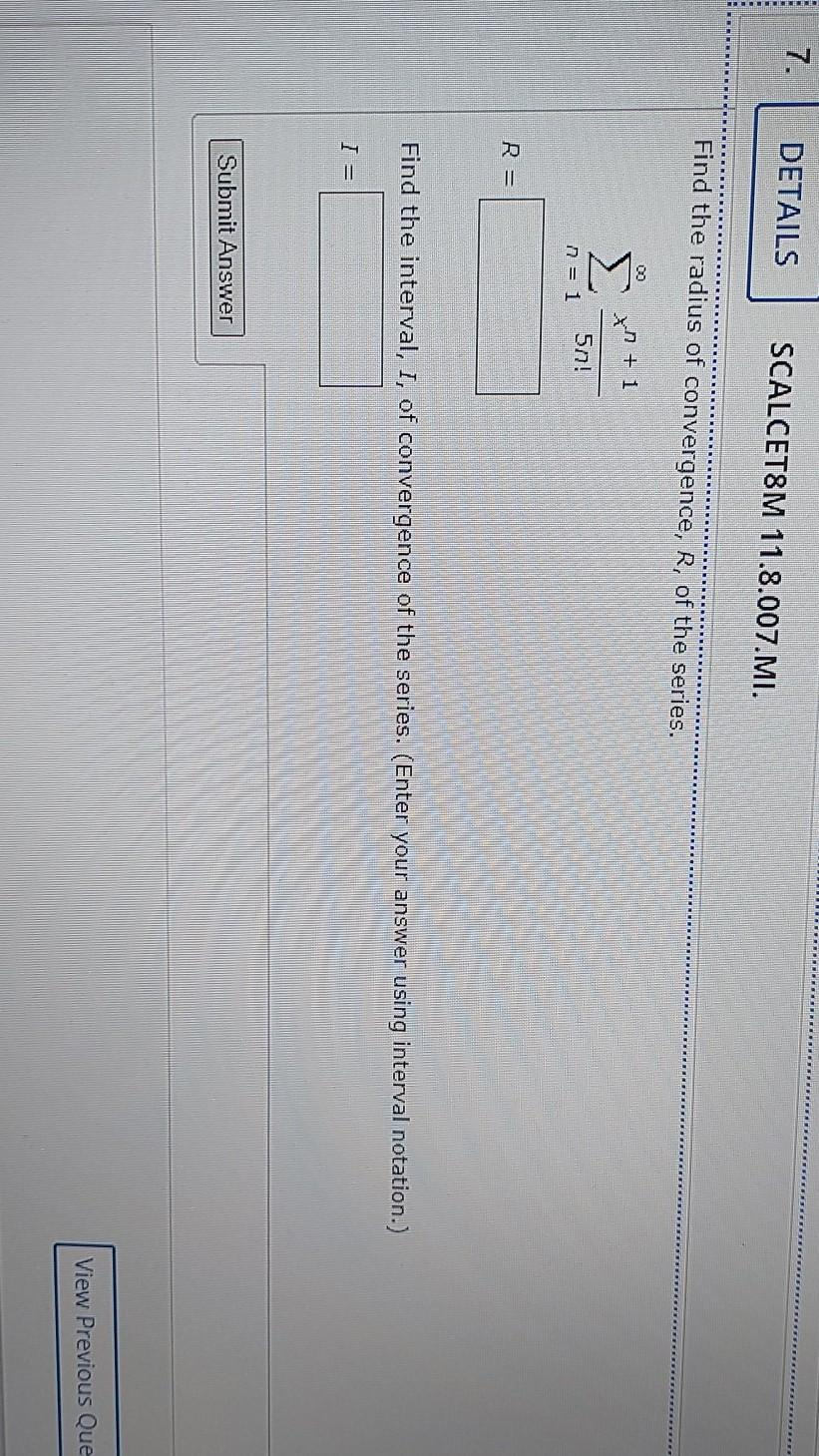### Create an Account

Home / Questions / 7. DETAILS SCALCET8M 11.8.007.MI. Find the radius of convergence, R, of the series. x + 1 ...

# 7. DETAILS SCALCET8M 11.8.007.MI. Find the radius of convergence, R, of the series. x + 1 50! n = 1 R = Find the interval, I, of convergence of the series. (Enter your answer using interval notation.)

7. DETAILS SCALCET8M 11.8.007.MI. Find the radius of convergence, R, of the series. x + 1 50! n = 1 R = Find the interval, I, of convergence of the series. (Enter your answer using interval notation.) I= Submit Answer View Previous QueJun 01 2021 View more View LessSubscribe To Get Solution